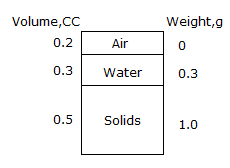# Civil Engineering - UPSC Civil Service Exam Questions - Discussion

31.

Consider the following statements in relation to the given sketch :
1. Soil is partially saturated at degree of saturation = 60%
2. Void ratio = 40%
3. Water content = 30%
4. Saturated unit weight = 1.5 g/cc
Of these statements :[A]. 1, 2 and 3 are correct [B]. 1, 3 and 4 are correct [C]. 2, 3 and 4 are correct [D]. 1, 2 and 4 are correct

Answer: Option A

Explanation:

No answer description available for this question.

 Mrinmoy Nath said: (Apr 2, 2017) The Void ratio must be 1.

 Chhaya said: (May 17, 2017) Yes, Mrinmoy Void ratio is 1 and the right answer is B.

 Hayat said: (Jul 25, 2017) No, Degree of saturation =60%. ("") e = 1 (X) w= 30% ("") Saturated unit weight = 1.3 g/cc (X).

 Shiva said: (Sep 21, 2017) Option B is correct.

 Dolly said: (Apr 11, 2018) The void ratio=volume of voids/volume of soil. e=.2/.5=.4 i.e=40%. How void ratio comes 1? Please explain.

 Skc said: (Sep 24, 2018) Vol Of voids= Va+ Vw. e = (Va+ Vw)/Vs= .5/.5= 1.

 Aseet Swami said: (Mar 17, 2020) 1) Soil is partially saturated at degree of saturation (S) = Vw/Vs = 0.3/0.5 = 60%. 2) Void ratio (e) = Vv/Vs = 0.2+0.3/0.5 = 100%. 3) Water content (w) = Ww/Ws = 0.3/1.0 = 30%. 4) Saturated unit weight (Ysat). Note: Each milliliter of water weighs 1gm. Hence, if the soil mass is to saturate the volume of water is the same as the weight of water. As a result weight of water is to be added is 0.2gms for full saturation. Ysat = 0.2+0.3+1.0/1 = 1.5 g/cc.

 Pooja Nikum said: (Jun 26, 2020) For Saturated unit weight = 1.5 g/cc. For fully saturated soil all the voids r filled with water, so total voids Vv= Va+Vw. And Total weight W= Ww+Ws= 0.3+1+0.2(It is the wt of water which is filled in 0.2 cc which is prior volume of air) and 0.2cc has 0.2g wt as given in ques that 0.3 cc has 0.3g wt. Ysat= W/V. =(0.3+1+0.2) /(0.2+0.3+0.5), = 1.5g/cc.

 Mahesh said: (May 1, 2021) Ysat = (.3+1)/(.2+.3+.5).

 Rahmat Ullah said: (Jun 10, 2021) e = volume of void / volume of solid = (0.2 + 0.3) / 0.5 = 1 x 100 = 100%. Sr = Vw / Vv = 0.3 / 0.5 = 0.6 x 100 = 60% w = Ww / Ws = 0.3 / 1 = 0.3 x 100 = 30 %. G = ( Sr x e) / w = (0.6 x 1) / 0.3 = 2. Ysat = Yw x (G + e) / (1 + e) = 1 x (2 + 1) / (1+1). = 1.5 g/cc. So, option B is Right.

#### Post your comments here:

Name *:

Email   : (optional)

» Your comments will be displayed only after manual approval.

#### Current Affairs 2021

Interview Questions and Answers